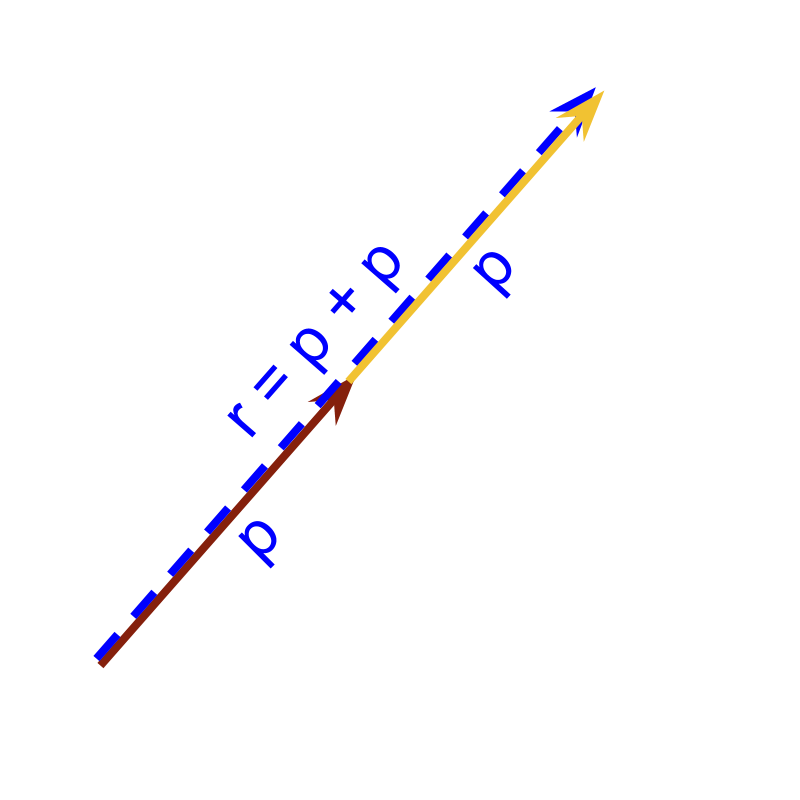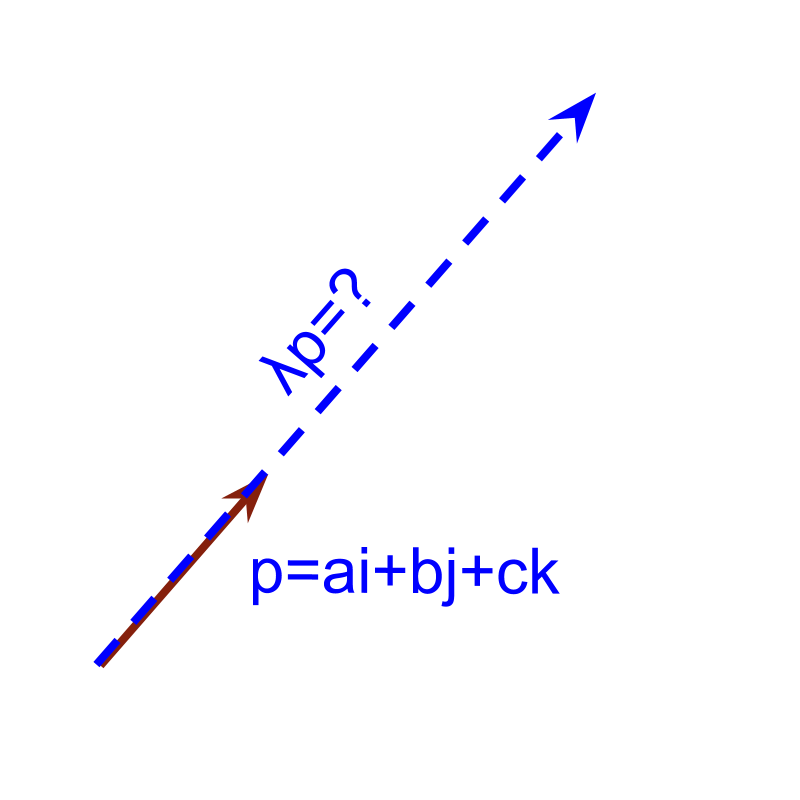maths > vector-algebra

what you'll learn...

Overview

»  Repeated addition is generalized to Multiplication of a vector by Scalar

»  Components are multiplied by scalar
→  $\stackrel{\to }{p}=ai+bj+ck$$\vec{p} = a i + b j + c k$
→  $\lambda \stackrel{\to }{p}=\lambda ai+\lambda bj+\lambda ck$$\lambda \vec{p} = \lambda a i + \lambda b j + \lambda c k$We have learned about addition of two or more vectors. Consider a vector that is added to itself. The result of the addition $\stackrel{\to }{p}+\stackrel{\to }{p}$$\vec{p} + \vec{p}$ is ' $=2×\stackrel{\to }{p}$$= 2 \times \vec{p}$'. If $\stackrel{\to }{p}=ai+bj+ck$$\vec{p} = a i + b j + c k$ then $\stackrel{\to }{p}+\stackrel{\to }{p}=2ai+2bj+2ck$$\vec{p} + \vec{p} = 2 a i + 2 b j + 2 c k$. This equals $2×\stackrel{\to }{p}$$2 \times \vec{p}$.Repeated addition can be generalized to multiplication of vector by a scalar. Scalar multiplier is denoted with $\lambda$$\lambda$ to identify differently to the component values $a,b,c$$a , b , c$. Note that the scalar multiplier and component values are real numbers.

$\stackrel{\to }{p}=ai+bj+ck$$\vec{p} = a i + b j + c k$
The result of $\lambda \stackrel{\to }{p}$$\lambda \vec{p}$ is '$\lambda ai+\lambda bj+\lambda ck$$\lambda a i + \lambda b j + \lambda c k$'.

Can a vector $\stackrel{\to }{p}$$\vec{p}$ be divided by a real number $\lambda$$\lambda$?
Yes. Division is inverse of multiplication.

If the given vector is $\stackrel{\to }{p}=ai+bj+ck$$\vec{p} = a i + b j + c k$ then
$\stackrel{\to }{p}÷\lambda$$\vec{p} \div \lambda$
$\quad \quad = \frac{1}{\lambda} \times \vec{p}$
Multiplication of Vector by a scalar: For any vector $\stackrel{\to }{p}=ai+bj+ck\in V$$\vec{p} = a i + b j + c k \in \mathbb{V}$ and scalar $\lambda \in ℝ$$\lambda \in \mathbb{R}$
$\lambda \stackrel{\to }{p}=\lambda ai+\lambda bj+\lambda ck$$\lambda \vec{p} = \lambda a i + \lambda b j + \lambda c k$